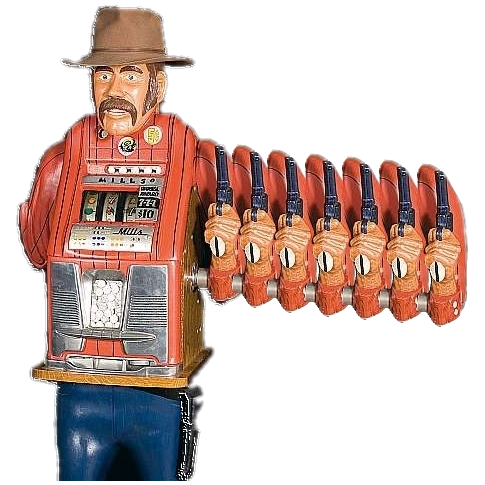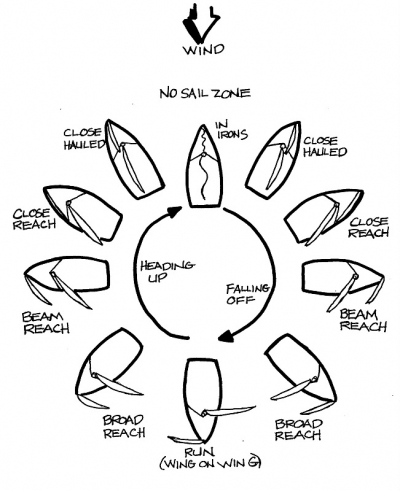# MCTS Based on Simple RegretAAAI 2012

## Multi-armed Bandits• • A set of $K$ arms.
• • Each arm can be pulled multiple times.
• • When the $i$th arm is pulled, a random reward $X_i$ is encountered.
• • Simple regret (SR): the reward of the last pull only is collected.
• • Cumulative regret (CR): all rewards are accumulated.

## UCB and UCT

• • UCB$(c)$ pulls arm $i$ that maximizes upper confidence bound $b_i$ on the reward: $b_i=\overline X_i+\sqrt {\frac {c \log (n)} {n_i}}$
• • UCB is nearly optimal in minimizing the cumulative regret.
• • UCT extends UCB to MCTS by invoking UCB at every node of a rollout.

## Metareasoning

• • A problem-solving agent can perform base-level actions from a known set $\{A_i\}$ .
• • Before committing to an action, the agent may perform a sequence of meta-level deliberation actions from a set $\{S_j\}$ .
• • At any given time there is a base-level action $A_\alpha$ that maximizes the agent's expected utility.
• • The value of information $VOI_j$ is the expected difference between the expected utilities of the new and the old selected base-level action after meta-level action $S_j$ is taken.
• • The agent selects a meta-level action that maximizes the VOI, or $A_\alpha$ if no meta-level action has positive VOI.

## Acknowledgments

• • IMG4 Consortium under the MAGNET program of the Israeli Ministry of Trade and Industry
• • Israel Science Foundation grant 305/09
• • Lynne and William Frankel Center for Computer Sciences
• • Paul Ivanier Center for Robotics Research and Production Management

## Experiments

• • SR+CR outperforms UCT.
• • SR+UCT($c$) is less dependent on tuning of the exploration factor $c$.

### Sailing Domain## Monte-Carlo Sampling in Trees

• • MCTS performs multiple rollouts to partially explore the search space.
• • At the current root node, the sampling is aimed at finding the first move to perform: minimizing the simple regret is more appropriate at the root node.
• • Deeper in the tree, minimizing cumulative regret results in a more precise estimate of the value of the state.
• • An improvement over UCT can be achieved by combining different sampling schemes on the first step and during the rest of a rollout.

## Main Results

### The SR+CR MCTS Scheme

• • Selects an action at the current root suitable for minimizing the simple regret.
• • Then selects actions according to UCB, that approximately minimizes the cumulative regret.

1 Rollout(node, depth=1) 2 if IsLeaf(node, depth) 3 return 0 4 else 5 if depth=1 then action $\gets$ FirstAction(node) 6 else action $\gets$ NextAction(node) 7 next $\gets$ NextState(node, action) 8 reward $\gets$ Reward(node, action, next) 9 + Rollout(next, depth+1) 10 UpdateStats(node, action, reward) 11 return reward

### Sampling for Simple Regret

1. $\varepsilon$-greedy sampling $\left(\varepsilon=\frac 1 2\right)$.
2. Modified version of UCB (optimized for simple regret).
3. VOI-aware sampling: $$VOI_\alpha\approx\frac {\overline X_\beta} {n_\alpha+1}\exp\left(-2(\overline X_\alpha - \overline X_\beta)^2 n_\alpha\right)$$ $$VOI_i\approx\frac {1-\overline X_\alpha} {n_i+1}\exp\left(-2(\overline X_\alpha - \overline X_i)^2 n_i\right),\; i\ne\alpha$$

## Contributions

• • Improved MCTS scheme — SR+CR.
• • SR+CR performs better than unmodified UCT.
• • VOI-aware sampling for minimizing simple regret.

## Future Work

• • Rational metareasoning in MCTS: theory and VOI estimates.
• • Better sampling for non-root nodes.
• • Application to Computer Go and other complex domains.